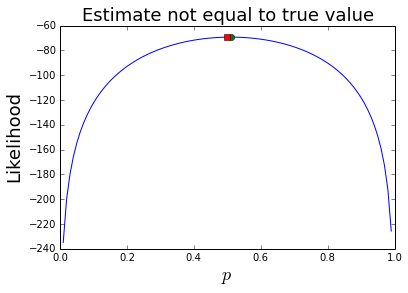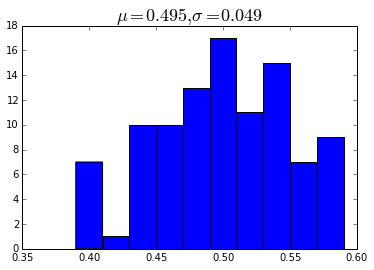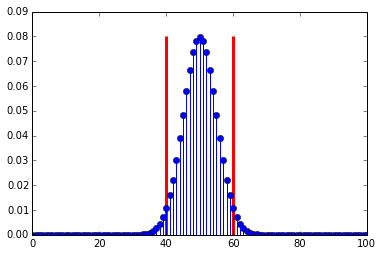## Maximum Likelihood Estimation¶

Maximum likelihood estimation is one of the key techniques employed in statistical signal processing for a wide variety of applications from signal detection to parameter estimation. In the following, we consider a simple experiment and work through the details of maximum likelihood estimation to ensure that we understand the concept in one of its simplest applications.

### Setting up the Coin Flipping Experiment¶

Suppose we have coin and want to estimate the probability of heads ($p$) for it. The coin is Bernoulli distributed:

$$\phi(x)= p^x (1-p)^{(1-x)}$$

where $x$ is the outcome, 1 for heads and 0 for tails. The $n$ independent flips, we have the likelihood:

$$\mathcal{L}(p|\mathbf{x})= \prod_{i=1}^n p^{ x_i }(1-p)^{1-x_i}$$

This is basically notation. We have just substituted everything into $\phi(x)$ under the independent-trials assumption.

The idea of maximum likelihood is to maximize this as the function of $p$ after plugging in all of the $x_i$ data. This means that our estimator, $\hat{p}$ , is a function of the observed $x_i$ data, and as such, is a random variable with its own distribution.

### Simulating the Experiment¶

We need the following code to simulate coin flipping.

In :
%matplotlib inline
from __future__ import division
from scipy.stats import bernoulli
import numpy as np

p_true=1/2 # this is the value we will try to estimate from the observed data
fp=bernoulli(p_true)

def sample(n=10):
'simulate coin flipping'
return fp.rvs(n)# flip it n times

xs = sample(100) # generate some samples


Now, we can write out the likelihood function using sympy

In :
import sympy
from sympy.abc import x, z
p=sympy.symbols('p',positive=True)

L=p**x*(1-p)**(1-x)
J=np.prod([L.subs(x,i) for i in xs]) # objective function to maximize


Below, we find the maximum using basic calculus. Note that taking the log of $J$ makes the maximization problem tractable but doesn't change the extrema.

In :
logJ=sympy.expand_log(sympy.log(J))
sol=sympy.solve(sympy.diff(logJ,p),p)

x=linspace(0,1,100)
plot(x,map(sympy.lambdify(p,logJ,'numpy'),x),sol,logJ.subs(p,sol),'o',
p_true,logJ.subs(p,p_true),'s',)
xlabel('$p$',fontsize=18)
ylabel('Likelihood',fontsize=18)
title('Estimate not equal to true value',fontsize=18)

C:\Python27\lib\site-packages\numpy\__init__.py:1: RuntimeWarning: divide by zero encountered in log
"""

Out:
<matplotlib.text.Text at 0xcb707f0>Note that our estimator $\hat{p}$ (red circle) is not equal to the true value of $p$ (green square), but it is at the maximum of the likelihood function. This may sound disturbing, but keep in mind this estimate is a function of the random data; and since that data can change, the ultimate estimate can likewise change. I invite you to run this notebook a few times to observe this. Remember that the estimator is a function of the data and is thus also a random variable, just like the data is.

Let's write some code to empirically examine the behavior of the maximum likelihood estimator using a simulation of multiple trials. All we're doing here is combining the last few blocks of code.

In :
def estimator_gen(niter=10,ns=100):
'generate data to estimate distribution of maximum likelihood estimator'
out=[]
x=sympy.symbols('x',real=True)
L=   p**x*(1-p)**(1-x)
for i in range(niter):
xs = sample(ns) # generate some samples from the experiment
J=np.prod([L.subs(x,i) for i in xs]) # objective function to maximize
logJ=sympy.expand_log(sympy.log(J))
sol=sympy.solve(sympy.diff(logJ,p),p)
out.append(float(sol.evalf()))
return out if len(out)>1 else out # return scalar if list contains only 1 term

etries = estimator_gen(100) # this may take awhile, depending on how much data you want to generate
hist(etries) # histogram of maximum likelihood estimator
title('$\mu=%3.3f,\sigma=%3.3f$'%(mean(etries),std(etries)),fontsize=18)

Out:
<matplotlib.text.Text at 0xcee5cd0>Note that the mean of the estimator ($\mu$) is pretty close to the true value, but looks can be deceiving. The only way to know for sure is to check if the estimator is unbiased, namely, if

$$\mathbb{E}(\hat{p}) = p$$

Because this problem is simple, we can solve for this in general noting that since $x=0$ or $x=1$, the terms in the product of $\mathcal{L}$ above are either $p$, if $x_i=1$ or $1-p$ if $x_i=0$. This means that we can write

$$\mathcal{L}(p|\mathbf{x})= p^{\sum_{i=1}^n x_i}(1-p)^{n-\sum_{i=1}^n x_i}$$

with corresponding log as

$$J=\log(\mathcal{L}(p|\mathbf{x})) = \log(p) \sum_{i=1}^n x_i + \log(1-p) \left(n-\sum_{i=1}^n x_i\right)$$

Taking the derivative of this gives:

$$\frac{dJ}{dp} = \frac{1}{p}\sum_{i=1}^n x_i + \frac{(n-\sum_{i=1}^n x_i)}{p-1}$$

$$\hat{p} = \frac{1}{ n} \sum_{i=1}^n x_i$$

This is our estimator for $p$. Up til now, we have been using sympy to solve for this based on the data $x_i$ but now we have it generally and don't have to solve for it again. To check if this estimator is biased, we compute its expectation:

$$\mathbb{E}\left(\hat{p}\right) =\frac{1}{n}\sum_i^n \mathbb{E}(x_i) = \frac{1}{n} n \mathbb{E}(x_i)$$

by linearity of the expectation and where

$$\mathbb{E}(x_i) = p$$

Therefore,

$$\mathbb{E}\left(\hat{p}\right) =p$$

This means that the esimator is unbiased. This is good news. We almost always want our estimators to be unbiased. Similarly,

$$\mathbb{E}\left(\hat{p}^2\right) = \frac{1}{n^2} \mathbb{E}\left[\left( \sum_{i=1}^n x_i \right)^2 \right]$$

and where

$$\mathbb{E}\left(x_i^2\right) =p$$

and by the independence assumption,

$$\mathbb{E}\left(x_i x_j\right) =\mathbb{E}(x_i)\mathbb{E}( x_j) =p^2$$

Thus,

$$\mathbb{E}\left(\hat{p}^2\right) =\left(\frac{1}{n^2}\right) n \left[ p+(n-1)p^2 \right]$$

So, the variance of the estimator, $\hat{p}$ is the following:

$$\sigma_\hat{p}^2 = \mathbb{E}\left(\hat{p}^2\right)- \mathbb{E}\left(\hat{p}\right)^2 = \frac{p(1-p)}{n}$$

Note that the $n$ in the denominator means that the variance asymptotically goes to zero as $n$ increases (i.e. we consider more and more samples). This is good news also because it means that more and more coin flips leads to a better estimate of the underlying $p$.

Unfortunately, this formula for the variance is practically useless because we have to know $p$ to compute it and $p$ is the parameter we are trying to estimate in the first place! But, looking at $\sigma_\hat{p}^2$, we can immediately notice that if $p=0$, then there is no estimator variance because the outcomes are guaranteed to be tails. Also, the maximum of this variance, for whatever $n$, happens at $p=1/2$. This is our worst case scenario and the only way to compensate is with more samples (i.e. larger $n$).

All we have computed is the mean and variance of the estimator. In general, this is insufficient to characterize the underlying probability density of $\hat{p}$, except if we somehow knew that $\hat{p}$ were normally distributed. This is where the powerful central limit theorem comes in. The form of the estimator, which is just a mean estimator, implies that we can apply this theorem and conclude that $\hat{p}$ is normally distributed. However, there's a wrinkle here: the theorem tells us that $\hat{p}$ is asymptotically normal, it doesn't quantify how many samples $n$ we need to approach this asymptotic paradise. In our simulation this is no problem since we can generate as much data as we like, but in the real world, with a costly experiment, each sample may be precious. In the following, we won't apply this theorem and instead proceed analytically.

### Probability Density for the Estimator¶

To write out the full density for $\hat{p}$, we first have to ask what is the probability that the estimator will equal a specific value and the tally up all the ways that could happen with their corresponding probabilities. For example, what is the probability that

$$\hat{p} = \frac{1}{n}\sum_{i=1}^n x_i = 0$$

This can only happen one way: when $x_i=0 \hspace{0.5em} \forall i$. The probability of this happening can be computed from the density

$$f(\mathbf{x},p)= \prod_{i=1}^n \left(p^{x_i} (1-p)^{1-x_i} \right)$$$$f\left(\sum_{i=1}^n x_i = 0,p\right)= \left(1-p\right)^n$$

Likewise, if $\lbrace x_i \rbrace$ has one $i^{th}$ value equal to one, then

$$f\left(\sum_{i=1}^n x_i = 1,p\right)= n p \prod_{i=1}^{n-1} \left(1-p\right)$$

where the $n$ comes from the $n$ ways to pick one value equal to one from the $n$ elements $x_i$. Continuing this way, we can construct the entire density as

$$f\left(\sum_{i=1}^n x_i = k,p\right)= \binom{n}{k} p^k (1-p)^{n-k}$$

where the term on the left is the binomial coefficient of $n$ things taken $k$ at a time. This is the binomial distribution and it's not the density for $\hat{p}$, but rather for $n\hat{p}$. We'll leave this as-is because it's easier to work with below. We just have to remember to keep track of the $n$ factor.

#### Confidence Intervals¶

Now that we have the full density for $\hat{p}$, we are ready to ask some meaningful questions. For example,

$$\mathbb{P}\left( | \hat{p}-p | \le \epsilon p \right)$$

Or, in words, what is the probability we can get within $\epsilon$ percent of the true value of $p$. Rewriting,

$$\mathbb{P}\left( p - \epsilon p \lt \hat{p} \lt p + \epsilon p \right) = \mathbb{P}\left( n p - n \epsilon p \lt \sum_{i=1}^n x_i \lt n p + n \epsilon p \right)$$

Let's plug in some live numbers here for our worst case scenario where $p=1/2$. Then, if $\epsilon = 1/100$, we have

$$\mathbb{P}\left( \frac{99 n}{200} \lt \sum_{i=1}^n x_i \lt \frac{101 n}{200} \right)$$

Since the sum in integer-valued, we need $n> 100$ to even compute this. Thus, if $n=101$ we have

$$\mathbb{P}\left( \frac{9999}{200} \lt \sum_{i=1}^{101} x_i \lt \frac{10201}{200} \right) = f\left(\sum_{i=1}^{101} x_i = 50,p\right)= \binom{101}{50} (1/2)^{50} (1-1/2)^{101-50} = 0.079$$

This means that in the worst-case scenario for $p=1/2$, given $n=101$ trials, we will only get within 1% of the actual $p=1/2$ about 8% of the time. If you feel disappointed, that only means you've been paying attention. What if the coin was really heavy and it was costly to repeat this 101 times? Then, we would be within 1% of the actual value only 8% of the time. Those odds are terrible.

Let's come at this another way: given I could only flip the coin 100 times, how close could I come to the true underlying value with high probability (say, 95%)? In this case we are seeking to solve for $\epsilon$. Plugging in gives,

$$\mathbb{P}\left( 50 - 50 \epsilon \lt \sum_{i=1}^{100} x_i \lt 50 + 50 \epsilon \right) = 0.95$$

which we have to solve for $\epsilon$. Fortunately, all the tools we need to solve for this are already in scipy.

In :
import scipy.stats

b=scipy.stats.binom(100,.5) # n=100, p = 0.5, distribution of the estimator \hat{p}

f,ax= subplots()
ax.stem(arange(0,101),b.pmf(arange(0,101))) # heres the density of the sum of x_i

g = lambda i:b.pmf(arange(-i,i)+50).sum() # symmetric sum the probability around the mean
print 'this is pretty close to 0.95:%r'%g(10)
ax.vlines( [50+10,50-10],0 ,ax.get_ylim() ,color='r',lw=3.)

this is pretty close to 0.95:0.95395593307062954

Out:
<matplotlib.collections.LineCollection at 0xd079630>The two vertical lines in the plot show how far out from the mean we have to go to accumulate 95% of the probability. Now, we can solve this as

$$50 + 50 \epsilon = 60$$

which makes $\epsilon=1/5$ or 20%. So, flipping 100 times means I can only get within 20% of the real $p$ 95% of the time in the worst case scenario (i.e. $p=1/2$).

In :
b=scipy.stats.bernoulli(.5) # coin distribution
xs = b.rvs(100) # flip it 100 times
phat = mean(xs) # estimated p

print abs(phat-0.5) < 0.5*0.20 # did I make it w/in interval 95% of the time?

True


Let's keep doing this and see if we can get within this interval 95% of the time.

In :
out=[]
b=scipy.stats.bernoulli(.5) # coin distribution
for i in range(500): # number of tries
xs = b.rvs(100) # flip it 100 times
phat = mean(xs) # estimated p
out.append(abs(phat-0.5) < 0.5*0.20 ) # within 20%

print 'Percentage of tries within 20 interval = %3.2f'%(100*sum(out)/float(len(out) ))

Percentage of tries within 20 interval = 96.20


Well, that seems to work. Now we have a way to get at the quality of the estimator, $\hat{p}$.

## Summary¶

In this section, we explored the concept of maximum likelihood estimation using a coin flipping experiment both analytically and numerically with the scientific Python tool chain. There are two key points to remember. First, maximum likelihood estimation produces a function of the data that is itself a random variable, with its own statistics and distribution. Second, it's worth considering how to analytically derive the density function of the estimator rather than relying on canned packages to compute confidence intervals wherever possible. This is especially true when data is hard to come by and the approximations made in the central limit theorem are therefore harder to justify.

This IPython notebook is available for download. I urge you to experiment with the calculations for different parameters. As always, corrections and comments are welcome!